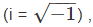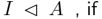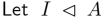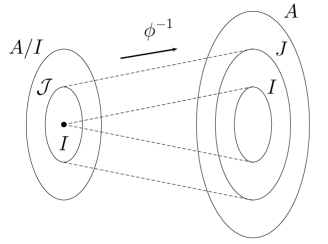Courses

# Rings, Ideals, Quotient Rings - Ring Theory Mathematics Notes | EduRev

## Algebra for IIT JAM Mathematics

Created by: Veda Institute

## Mathematics : Rings, Ideals, Quotient Rings - Ring Theory Mathematics Notes | EduRev

The document Rings, Ideals, Quotient Rings - Ring Theory Mathematics Notes | EduRev is a part of the Mathematics Course Algebra for IIT JAM Mathematics.
All you need of Mathematics at this link: Mathematics

A ring A is a set with + , • such that

 (1) (A, +) is an abelian group;(2) (A, •) is a semigroup;(3) • distributes over + on both sides.(4) (∀x, y ∈ A) x • y = y • x(5) (∃1 ∈ A)(∀x ∈ A) 1•x = x•1 = x .

In this course all rings A are commutative, that is, and have an identity element 1 (easily seen to be unique)

 If 1 = 0 then A = {0} (easy to see), called the zero ring.

Multiplication will be denoted by juxtaposition, and simple facts used without comment, such as

 (∀x, y ∈ A)x 0 = 0 ,(−x)y = x(−y) = −(xy) ,(−x)(−y) = xy

Call a subset S of a ring A a subring if

 (i) 1 ∈ S ;(ii) (∀x, y ∈ S ) x + y , xy , −x ∈ S .

Condition (ii) is easily seen to be equivalent to

 (ii)′ (∀x, y ∈ S ) x − y , xy ∈ S .

 Note: In other contexts authors replace the condition 1 ∈ S by S ≠ ∅ (which is not equivalent!).

Examples:

(1) Z is the only subring of Z .

(2) Z is a subring of Q , which is a subring of R , which is a subring of C .

(3) Z[i] = { a + bi | a, b ∈ Z }the ring of Gaussian integers is a subring of C

(4) Zn = { 0, 1, . . . , n − 1}

with addition and multiplication mod n .

(Alternatively Zn may be deﬁned to be the quotient ring Z/nZ , deﬁned below)

(5) R any ring, x an indeterminate. Put

R[[x]] = {a0 + a1x + a2x2 + . . . | a0, a1, . . . ∈ R} , the set of formal power series over R , which becomes a ring under addition and multiplication of power series. Important subring:

R[x] = {a0 + a1x + . . . +anxn | n ≥ 0 , a0, a1, . . . , an ∈ R}

the ring of polynomials over R .

Call a mapping f : A → B (where A and B are rings) a ring homomorphism if

 (a) f (1) = 1 ;(b) (∀x, y ∈ A) f (x + y) = f (x) + f (y)andf (xy) = f (x)f (y) ,

in which case the following are easily checked:

 (i) f (0) = 0 ;(ii) (∀x ∈ A) f (−x) = −f (x) ;(iii) f (A) = {f (x) | x ∈ A} , the image of f is a subring of B ;(iv) Composites of ring hom’s are ring hom’s.

An isomorphism is a bijective homomorphism, say f : A → B , in which case we write

A ≌ B or f : A ≌ B.

It is easy to check that

 ≌ is an equivalence relation.

A nonempty subset I of a ring A is called an ideal, written(i) (∀x, y ∈ I ) x + y , −x ∈ I [clearly equivalent to (i)′ (∀x, y ∈ I ) x − y ∈ I ]:(ii) (∀x ∈ I )(∀y ∈ A) xy ∈ I .

In particular I is an additive subgroup of A , so we can form the quotient group the group of cosets of I ,

A/I = { I + a | a ∈ A} ,

with addition deﬁned by, for a, b ∈ A , (I + a) + (I + b) = I + (a + b) .

Further A/I forms a ring by deﬁning, for a, b ∈ A ,

(I + a) (I + b) = I + (a b) .

 Veriﬁcation of the ring axioms is straightforward:

— only tricky bit is ﬁrst checking multiplication is well-deﬁned:

If I + a = I + a′ and I + b = I + b′ then

a − a′ , b − b′ ∈ I ,

so

ab − a′b′ = ab − ab′ + ab′ − a′b′

= a(b − b′) + (a − a′)b′ ∈ I

yielding I + ab = I + a′b′ .

We call A/I a quotient ring.

The mapping

φ : A → A/I , x → I + x

is clearly a surjective ring homomorphism, called the natural map, whose kernel is

ker φ = {x ∈ A | I + x = I} = I .

Thus all ideals are kernels of ring homomorphisms.

The converse is easy to check, so

 kernels of ring homomorphisms with domain A are precisely ideals of A .

The following important result is easy to verify:

 Fundamental HomomorphismTheorem: If f : A → B is a ring homomorphism with kernel I and image C thenA/I ≌ C .

 Proposition:and φ : A → A/I be the natural map. Then(i) ideals J of A/I have the form J = J/I = {I + j | j ∈ J} for some J such that I ⊆ J  A ; (ii) φ−1 is an inclusion-preserving bijection between ideals of A/I and ideals of A containing I .Example: The ring Zn = {0, 1, . . . , n − 1} with mod n arithmetic is isomorphic to Z/nZ : follows from the Fundamental Homomorphism

Theorem, by observing that the mapping f : Z → Zn where

f (z) = remainder after dividing z by n is a ring homomorphism with image Zn and kernel nZ.

Offer running on EduRev: Apply code STAYHOME200 to get INR 200 off on our premium plan EduRev Infinity!

,

,

,

,

,

,

,

,

,

,

,

,

,

,

,

,

,

,

,

,

,

,

,

,

,

,

,

;